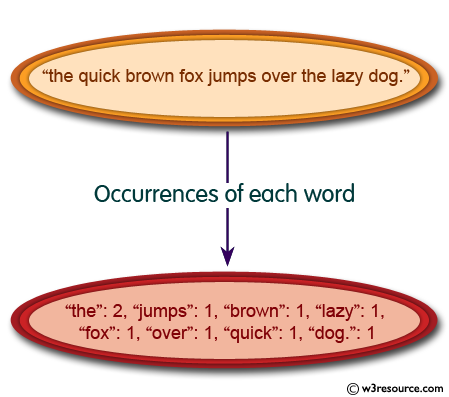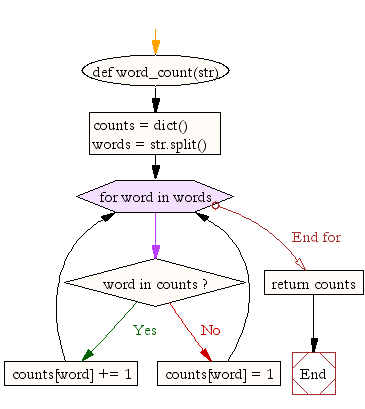﻿ Python: Count the occurrences of each word in a given sentence - w3resource# Python: Count the occurrences of each word in a given sentence

## Python String: Exercise-12 with Solution

Write a Python program to count the occurrences of each word in a given sentence.Sample Solution:-

Python Code:

``````def word_count(str):
counts = dict()
words = str.split()

for word in words:
if word in counts:
counts[word] += 1
else:
counts[word] = 1

return counts

print( word_count('the quick brown fox jumps over the lazy dog.'))
```
```

Sample Output:

```{'the': 2, 'jumps': 1, 'brown': 1, 'lazy': 1, 'fox': 1, 'over': 1, 'quick': 1, 'dog.': 1}
```

Flowchart:## Visualize Python code execution:

The following tool visualize what the computer is doing step-by-step as it executes the said program:

Python Code Editor:

Have another way to solve this solution? Contribute your code (and comments) through Disqus.

What is the difficulty level of this exercise?

Test your Programming skills with w3resource's quiz.

﻿

## Python: Tips of the Day

Check if a given key already exists in a dictionary:

In is the intended way to test for the existence of a key in a dict.

```d = {"key1": 10, "key2": 23}

if "key1" in d:
print("this will execute")

if "nonexistent key" in d:
print("this will not")
```

If you wanted a default, you can always use dict.get():

```d = dict()

for i in range(100):
key = i % 10
d[key] = d.get(key, 0) + 1
```

and if you wanted to always ensure a default value for any key you can either use dict.setdefault() repeatedly or defaultdict from the collections module, like so:

```from collections import defaultdict

d = defaultdict(int)

for i in range(100):
d[i % 10] += 1
```

but in general, the in keyword is the best way to do it.

Ref: https://bit.ly/2XPMRyz